Show Question
Math and science::Analysis

# 18 analysis theorems

The following propositions about an ordered field $$R$$ (and about associated structures such as $$[a,b]=[a,b]_R=\{x \in R:\ a \leq x \leq b\}$$) are true when the ordered field $$R$$ is taken to be $$\mathbb{R}$$, the field of real numbers.

1. The Dedekind Completeness Property (Supremum)
Suppose $$S$$ is a nonempty subset of $$R$$ that is bounded above. Then there exists a number $$c$$ that is an upper bound of $$S$$ such that every upper bound of $$S$$ is greater than or equal to $$c$$.
We refer to this as completeness.
2. The Archimedean Property
For every $$x > 0, x \in R$$ there exists $$n \in \mathbb{N}$$ with $$n > x$$. Equivalently, for every $$x > 0, x \in R$$ there exists $$n \in \mathbb{N}$$ with $$1/n < x$$.
Doesn't imply completeness.
3. The Cut Property
Suppose $$A$$ and $$B$$ are nonempty disjoint subsets of $$R$$ whose union is all of $$R$$, such that every element of $$A$$ is less than every element of $$B$$. Then there exists a cutpoint $$c \in R$$ such that every $$x < c$$ is in $$A$$ and every $$x>c$$ is in $$B$$. (Or, if you prefer: Every $$x \in A$$ is $$\leq c$$, and every $$x \in B$$ is $$\geq c$$. It's easy to check that the two versions are equivalent.) Since this property may be unfamiliar, we remark that the Cut Property follows immediately from Dedekind completeness (take $$c$$ to be the least upper bound of $$A$$).
Does imply completeness.
4. Topological Connectedness
Say $$S \subseteq R$$ is open if for every $$x$$ in $$S$$ there exists $$\epsilon > 0$$ so that every $$y$$ with $$|y-x| < \epsilon$$ is also in $$S$$. Then there is no way to express $$R$$ as a union of two disjoint nonempty open sets. That is, if $$R = A \cup B$$ with $$A,B$$ nonempty and open, then $$A \cap B$$ is nonempty.
Does imply completeness.
5. The Intermediate Value Property
If $$f$$ is a continuous function from $$[a,b]$$ to $$R$$, with $$f(a) < 0$$ and $$f(b) > 0$$, then there exists $$c$$ in $$(a,b)$$ with $$f(c) = 0$$.
Does imply completeness.
6. The Bounded Value Property
If $$f$$ is a continuous function from $$[a,b]$$ to $$R$$, then there exists $$B$$ in $$R$$ with $$f(x) \leq B$$ for all $$x$$ in $$[a,b]$$.
Doesn't imply completeness.
7. The Extreme Value Property
If $$f$$ is a continuous function from $$[a,b]$$ to $$R$$, then there exists $$c$$ in $$[a,b]$$ with $$f(x) \leq f(c)$$ for all $$x$$ in $$[a,b]$$.
Does imply completeness.
8. The Mean Value Property
Suppose $$f: [a,b] \rightarrow R$$ is continuous on $$[a,b]$$ and differentiable on $$(a,b)$$. Then there exists $$c$$ in $$(a,b)$$ such that $$f'(c) = (f(b)-f(a))/(b-a)$$.
Does imply completeness.
9. The Constant Value Property
Suppose $$f: [a,b] \rightarrow R$$ is continuous on $$[a,b]$$ and differentiable on $$(a,b)$$, with $$f'(x) = 0$$ for all $$x$$ in $$(a,b)$$. Then $$f(x)$$ is constant on $$[a,b]$$.
Does imply completeness.
10. The Convergence of Bounded Monotone Sequences
Every monotone increasing (or decreasing) sequence in $$R$$ that is bounded converges to some limit.
Does imply completeness.
11. The Convergence of Cauchy Sequences
Every Cauchy sequence in $$R$$ is convergent.
Doesn't imply completeness.
12. The Fixed Point Property for Closed Bounded Intervals
Suppose $$f$$ is a continuous map from $$[a,b] \subset R$$ to itself. Then there exists $$x$$ in $$[a,b]$$ such that $$f(x) = x$$.
Does imply completeness.
13. The Contraction Map Property
Suppose $$f$$ is a map from $$R$$ to itself such that for some constant $$c < 1$$, $$|f(x) - f(y)| \leq c|x-y|$$ for all $$x,y$$. Then there exists $$x$$ in $$R$$ such that $$f(x) = x$$.
Does imply completeness.
14. The Alternating Series Test
If $$a_1 \geq a_2 \geq a_3 \geq \dots$$ and $$a_n \rightarrow 0$$, then $$\sum_{n=1}^{\infty} (-1)^n a_n$$ converges.
Doesn't imply completeness.
15. The Absolute Convergence Property
If $$\sum_{n=1}^\infty |a_n|$$ converges in $$R$$, then $$\sum_{n=1}^\infty a_n$$ converges in $$R$$.
Doesn't imply completeness.
16. The Ratio Test
If $$|a_{n+1}/a_n| \rightarrow L$$ in $$R$$ as $$n \rightarrow \infty$$, with $$L < 1$$, then $$\sum_{n=1}^{\infty} a_n$$ converges in $$R$$.
Does imply completeness.
17. The Shrinking Interval Property
Suppose $$I_1 \supseteq I_2 \supseteq \dots$$ are bounded closed intervals in $$R$$ with lengths decreasing to 0. Then the intersection of the $$I_n$$'s is nonempty.
Doesn't imply completeness.
18. The Nested Interval Property
Suppose $$I_1 \supseteq I_2 \supseteq \dots$$ are bounded closed intervals in $$R$$. Then the intersection of the $$I_n$$'s is nonempty. (Another name for this property is "spherical completeness''.)
Doesn't imply completeness.

It's amazing to realize than many of these properties are equivalent to the supremum property, which is usually introduced first. It is also interesting to see which of these properties are not sufficient to imply completeness; and yet they are sufficient in the presence of the Archimedean property.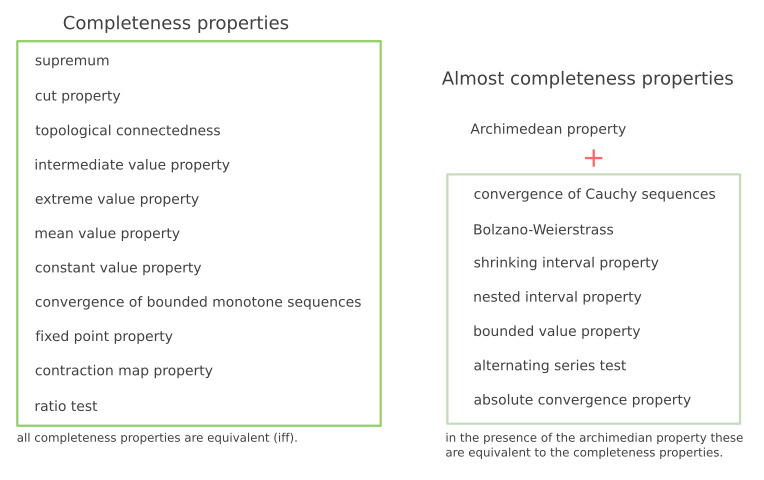The original text has short proofs and interactions for each of these. An exerpt: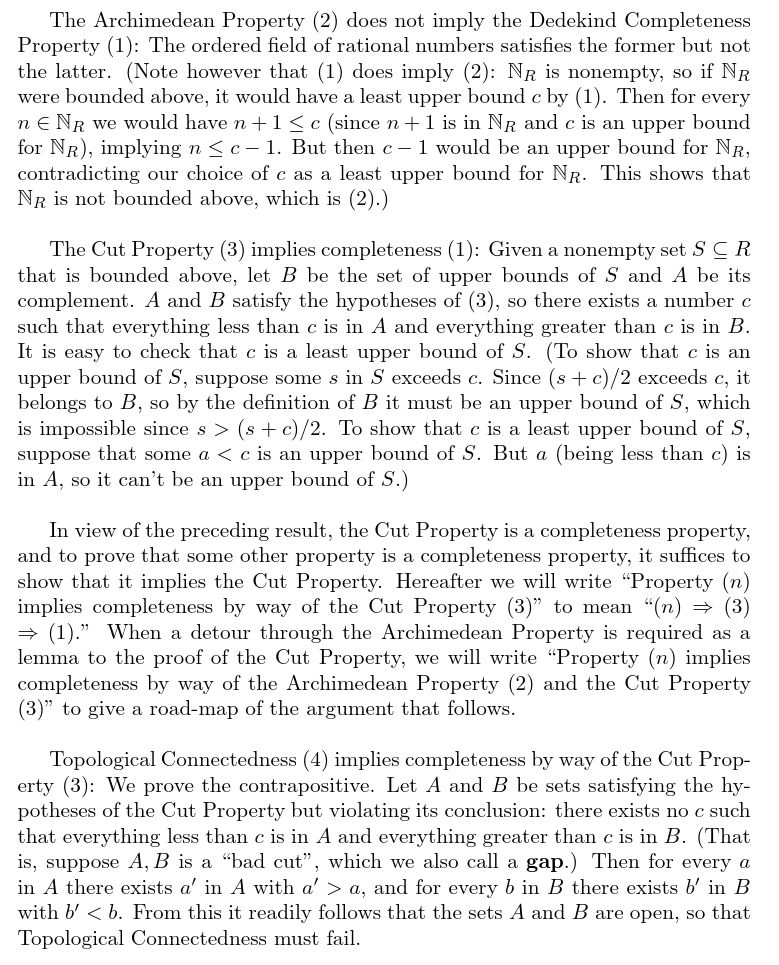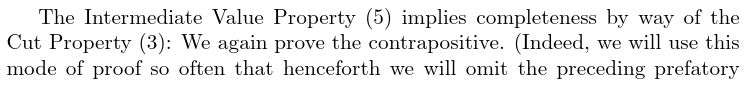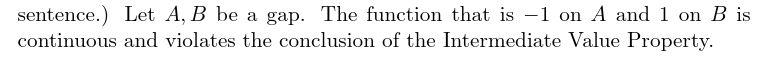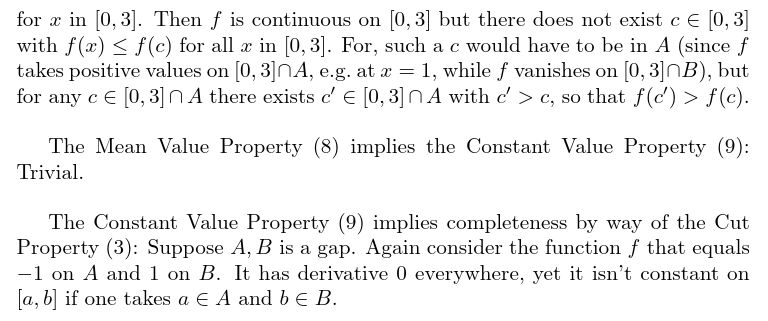#### Source

James Propp, "Real Analysis in Reverse"
https://arxiv.org/pdf/1204.4483.pdf